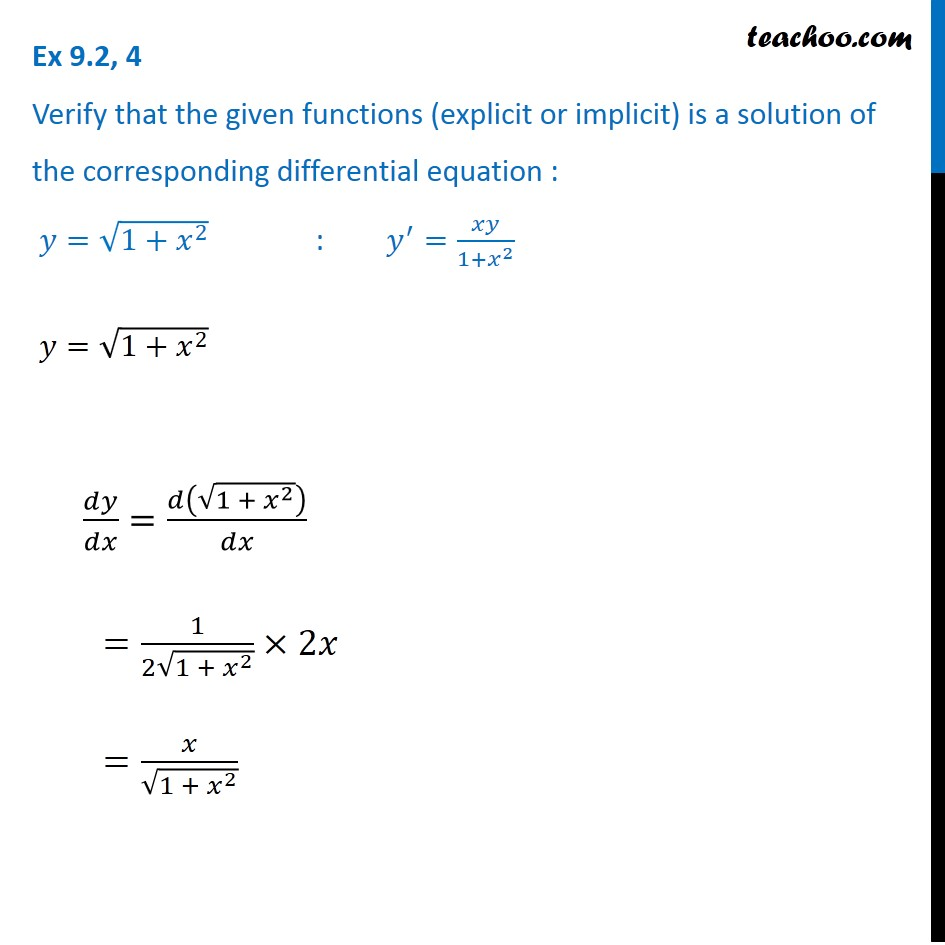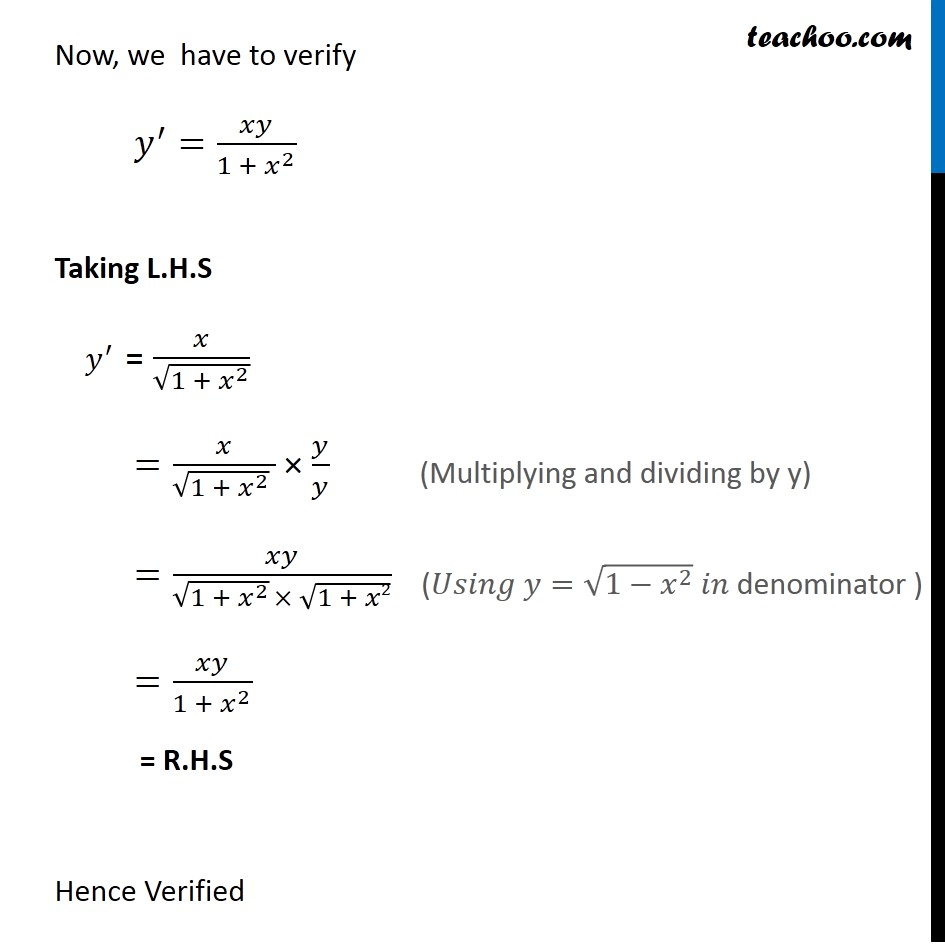Ex 9.2

Chapter 9 Class 12 Differential Equations
Serial order wiseLearn in your speed, with individual attention - Teachoo Maths 1-on-1 Class

### Transcript

Ex 9.2, 4 Verify that the given functions (explicit or implicit) is a solution of the corresponding differential equation : 𝑦=√(1+𝑥^2 ) : 𝑦^′=𝑥𝑦/(1+𝑥^2 ) 𝑦=√(1+𝑥^2 ) 𝑑𝑦/𝑑𝑥=𝑑(√(1 + 𝑥^2 ))/𝑑𝑥 =1/(2√(1 + 𝑥^2 ))×2𝑥 =𝑥/√(1 + 𝑥^2 ) Now, we have to verify 𝑦^′=𝑥𝑦/(1 + 𝑥^2 ) Taking L.H.S 𝑦^′ = 𝑥/√(1 + 𝑥^2 ) =𝑥/(√(1 + 𝑥^2 ) ) × 𝑦/𝑦 =𝑥𝑦/(√(1 + 𝑥^2 ) × √(1 + 𝑥2)) =𝑥𝑦/(1 + 𝑥^2 ) = R.H.S Hence Verified (Multiplying and dividing by y) (𝑈𝑠𝑖𝑛𝑔 𝑦=√(1−𝑥^2 ) 𝑖𝑛 denominator )